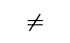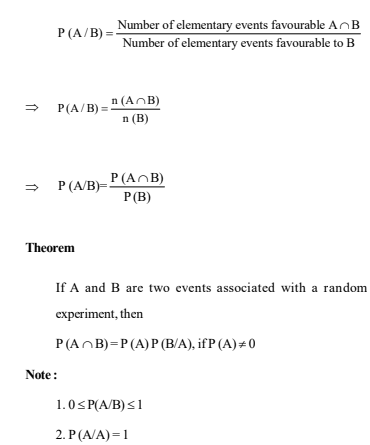## Conditional Probability

Let A and B be two events associated with a random

experiment. Then, the probability of occurrence of event A

under the condition that B has already occurred and

P (B)0, is called the conditional probability and it is denoted

by P (A/B). Thus, we have

P (A/B) = Probability of occurence of A given that B has